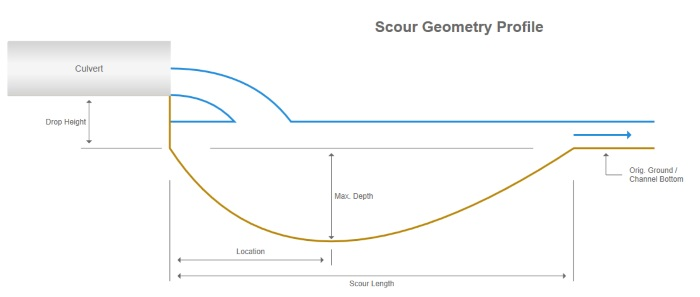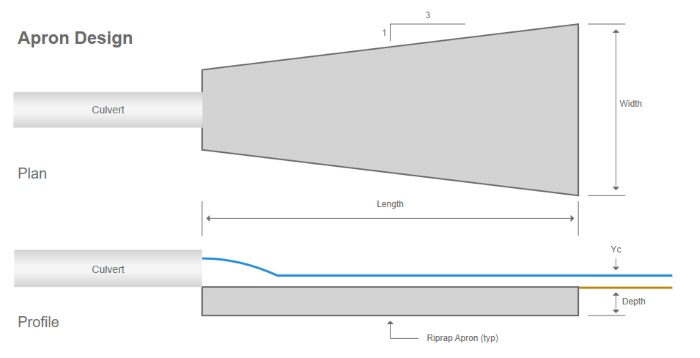1. Home
2. Computational Methods
3. Estimating Culvert Scour

# Estimating Culvert Scour

Culvert Studio can estimate local scour at the outlet of your culverts. Scour is based on discharge, culvert shape, soil type, duration of flow, culvert slope, drop height (height above the natural bed) and tailwater depth.

The software closely follows the computational procedures described in Hydraulic Engineering Circular 14.

The software closely follows the computational procedures described in Hydraulic Engineering Circular 14 for scour and apron design. The equations are based on tests that were conducted to determine maximum scour for the given condition and therefore represent what might be termed worst-case scour geometries.The methods are divided into two soil types:

1. Cohesionless
2. Cohesive

Each category has unique, but minimal, input requirements. The procedures however are quite lengthy and involve complex equations, terms, tables and assumptions. These procedures are omitted from this text due to their length. Please reference HEC-14 for complete details.

Time Duration
Please note that Culvert Studio assumes a fixed time duration of 30 minutes. Tests indicate that approximately 2/3 to 3/4 of the maximum scour depth occurs in the first 30 minutes of the flow duration.

## Riprap Apron Design

The most commonly used device for outlet protection is a riprap apron. An example schematic of an apron is shown below.They are constructed of riprap or grouted riprap at a zero grade for a distance that is related to the culvert pipe diameter. These aprons do not dissipate significant energy except through increased roughness for a short distance. However, they do serve to spread the flow helping to transition to the natural drainage way or to sheet flow where no natural drainage way exists.

The calculation procedure is fairly simple. The required riprap rock size, D50, is first computed. The apron dimensions are then pulled from a table.

The following equation is used to determine the Apron rock size, D50:Where:

D50 = required riprap size in ft (m)
Q = discharge in cfs (cms)
D = culvert rise (diameter) in ft (m)
Tw = tailwater depth in ft (m)
g = acceleration due to gravity

Tailwater depth is limited to between 0.4D and 1.0D. For example, if Tw is less than 0.4D then Tw = 0.4D.

D is subject to an adjustment when the flow Depth is below Critical Depth. The adjusted D, D’ = (D + Depth) / 2.

Once D50 is determined, it is converted to whole inches (mm). The Length and Depth dimensions are then taken from the following table:

ClassD50D50 (mm)Apron LengthApron Depth
151254D3.5D50
261504D3.3D50
3102505D2.4D50
4143506D2.2D50
5205007D2.0D50
6225508D2.0D50

The Apron Width is computed using a 3:1 flare as shown in the example schematic above.

### Apron Length Extension

If a Drop Height value greater than zero is entered and the starting Tw is not based on a Known Elevation or a Channel, Culvert Studio will use projectile motion equations to determine the location of where the water surface contacts the bed.

Culvert Studio will use projectile motion equations to determine the location of where the water surface contacts the bed

This horizontal distance is added to the computed apron length to insure the total required length is being utilized.### Downstream Apron Velocity

Under free-fall conditions at the culvert outlet, the downstream water surface depth on the apron is computed as critical depth, Yc, while using the computed Apron Width. The final velocity is based on this downstream depth.

If a Known Elevation is used as the Starting Tw, the velocity is based on the known Tw depth, that is, Known Elevation – Invert Elevation + Drop height.

If a Channel was used as the Starting Tw, the Channel’s velocity will be assumed.

Only the flow from the culvert(s) is used. Overtopping flow is not considered in computing this velocity.